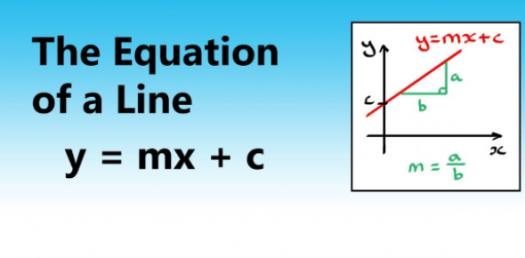# Algebra: Can You Write The Equation Of A Line?

4 Questions | Total Attempts: 678SettingsCan you formulate the equation of a line? Can you write the linear equation in standard form? The equation of a line is typically written with numbers and symbols. Do you know that two points a line passes through, you can find the equation of a line? Take this quiz and learn your algebra!

Related Topics
• 1.
Write the equation of the following line.
• A.

Y = 1/2x

• B.

Y = 2/1x

• C.

Y = 1/2x + 2

• D.

Y = 2/1x + 2

• 2.
Write the equation of the following line.
• A.

Y = -6

• B.

X = -6

• C.

Y = 0x + (-6)

• D.

X = 6

• 3.
Write the equation of the following line.
• A.

Y =0x + (-3)

• B.

X = -3

• C.

Y = 3

• 4.
Write the equation of the following line.
• A.

Y = -3/7x

• B.

Y = -7/3x

• C.

Y = -7/3x +3

• D.

Y = -3/7x + 3